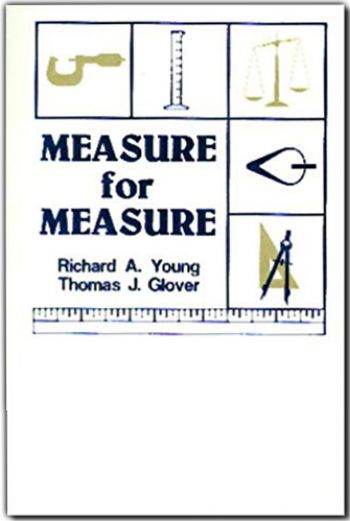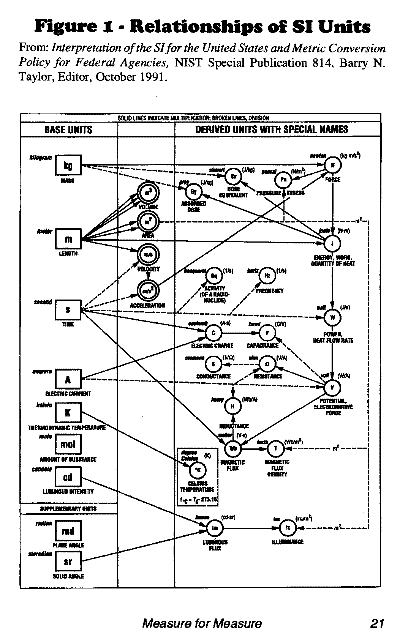Measure for Measure Softbound WhiteDescriptionMeasure for Measure is the most complete conversion factor reference on the market today and is an excellent reference for all engineers, scientists, teachers and students.Measure for Measure makes explicit distinctions between units of force and units of mass. For example, in the inch-pound system, units of mass and force are easily confused. Does the unit "pound" mean "pound-mass" or "pound-force"? Measure for Measure eliminates this confusion by attaching the modifier "-force" to all inch-pound force units. In Measure for Measure, the unit "pound-force" is converted only to units of force (i.e., dyne, poundal, pond, kilogram-force, newton, etc.) and the unit "pound" is converted only to units of mass (i.e., cental, slug, grain, ton, kilogram, etc.)Measure for Measure offers conversion factors that are more precise than those presented in other sources. For example, most sources give the energy conversion 1 BTU (international) = 1,055.1 joules. While this conversion factor is correct, it is not apparent to the user that it has been rounded upward from the exact definition, which is 1 BTU (international)= 1,055.05585262 joules. In Measure for Measure this conversion factor is given as "1 BUT (international) = 1,055.0559 joules," at least two significant digits more than other sources. Measure for Measure uses exact definitions wherever possible in calculating conversion factors, leaving it to users to round conversion factors to suit their needs.Measure for Measure carefully distinguishes between units with the same name by using an extensive list of modifiers. For example, the BTU is the amount of energy required to raise one pound of water through a temperature change of 1°F. The BTU is, therefore, sensitive to the starting temperature and volume of mass of water. For this reason, the BTU has been defined or adjusted at least nine times in the past 120 years. Despite this history of change, some conversion factor sources list only one BTU, presumably the most current, while other sources list two or three different BTUs. Measure for Measure presents nine different BTUs, ranging from "1 BTU (thermochemical)= 1,054.3500 joules" to 1 BTU (3.9°C)= 1,059.6700 joules."Measure for Measure is the most accurate conversion factor reference on the market today. Many hundreds of staff hours were used to check and verify each conversion factor in Measure for Measure. While some errors are inevitable in a work of this magnitude, the authors are confident that the number of errors is significantly less than in any other conversion source. Measure for Measure contains over 11,200 conversion factors for ancient and modern units from other countries, many more conversion factors than any other source.Measure for Measure is an outstanding reference for historians, bible scholars, Scrabble® players and crossword puzzle fanatics. Only Measure for Measure is your one source to find "1 aam (Germany) = 155 liters," "1 dimerlie (Romania) = 24.6 liters," "1 pied de perche (UK) = 279 millimeters," and 1 zuz (Hebrew, ancient) = 3.585 grams."Price: \$15.95